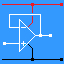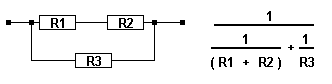RANDOM PAGE

SITE SEARCH

LOG
IN

HELP

# Resistors in Parallel

This is the AQA version closing after June 2019. Visit the the version for Eduqas instead.

Components connected in Parallel are wired together as shown in the diagram.For three resistors ...

Rtotal = 1 / ( 1 / R1 + 1 / R2 + 1 / R3 )

For just two resistors ...

Rtotal = 1 / ( 1 / R1 + 1 / R2 )

You need to be good at fractions or skilled with your calculator!

On a calculator, to get Rtotal press these keys ... 1 / ( 1 / R1 + 1 / R2 ) =

## Combined Series and Parallel

Problems like this need to be solved in stages by breaking the problem into simpler parts.

In the diagram below, add R1 + R2 to work out the series resistors.Then use the parallel resistors formula to work out R3 in parallel with the result of (R1 + R2).

## Another Series and Parallel Calculation

In the next example, work out each of the parallel resistors first and then add the two answers together.(R1 and R2) in parallel added to (R3 and R4) in parallel.

reviseOmatic V3     Contacts, ©, Cookies, Data Protection and Disclaimers Hosted at linode.com, London